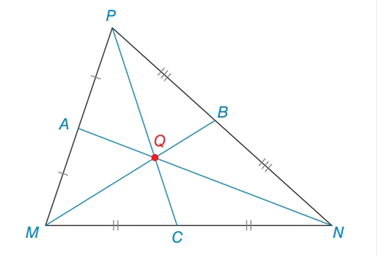Chapter 7.2, Problem 30E### Elementary Geometry for College St...

6th Edition
Daniel C. Alexander + 1 other
ISBN: 9781285195698

#### Solutions

Chapter
Section### Elementary Geometry for College St...

6th Edition
Daniel C. Alexander + 1 other
ISBN: 9781285195698
Textbook Problem
12 views

# In Δ M N P for Exercise 29, medians M B ¯ , N A ¯ and P C ¯ intersect at centroid Q .a) Find Q B If M Q = 8.2 .b) Find P Q if Q C = 7 2 . c). Find A N if A Q = 4.6Exercises 27, 28

To determine

(a)

To find:

The QB in the given triangle MNP.

Explanation

Definition:

The three medians of a triangle are congruent at a point that is two-thirds the distance from any vertex to the midpoint of the opposite side.

Given:

In ΔMNP, medians MB¯, NA¯ and PC¯ intersect at centroid Q. If MQ=8.2.

Calculation:

The point of concurrence Q for the three medians of a triangle is known as centroid of the triangle. In ΔMNP, medians MB¯, NA¯ and PC¯ intersect at centroid Q

To determine

(b)

To find:

The PQ in the given triangle MNP.

To determine

(c)

To find:

The AN in the given triangle MNP.

### Still sussing out bartleby?

Check out a sample textbook solution.

See a sample solution

#### The Solution to Your Study Problems

Bartleby provides explanations to thousands of textbook problems written by our experts, many with advanced degrees!

Get Started